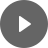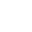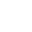10.2 Simultaneous Linear - StudyNinja
Select Page

HSC Standard Maths Resources

Browse: 1. Home  »  10. Linear Relationship »  10.2 Simultaneous Linear

10.2 Simultaneous Linear

So far, we looked at one linear equation; there are times when you are provided with two linear equations and you have to look for a solution. By solution we mean values of x and y that are common in both equations. There are two ways of solving these equations: Graphically and Algebraically.

Example 1

Adam has a business of selling cars. The following linear equations define his revenue and costs.

Revenue = 27,000 × no. of cars sold

Cost = 150,000 + 12,000 × no. of cars sold

What is the break-even point?

Get access to 20 Mock Exams with over 700 exam-style questions for HSC Standard Maths.

You might find the following videos helpful related to this section:5C Solving Simultaneous Equations Graphically

by Mr Bodgers (click to view channel)

Solving simultaneous equations graphically by first writing up a table of values.5D Solving Simultaneous Equations Algebraically (2 of 3)

by Mr Bodgers (click to view channel)

4 examples that show you how to solve simultaneous equations using the substitution method.5D Solving Simultaneous Equations Algebraically (2 of 3)

by Mr Bodgers (click to view channel)

4 examples that show you how to solve simultaneous equations using the substitution method.5D Solving Simultaneous Equations Algebraically (3 of 3)

by Mr Bodgers (click to view channel)

4 examples that show you how to solve simultaneous equations using the elimination method.5E Solving Problems Using Simultaneous Equations (1 of 2)

by Mr Bodgers (click to view channel)

Solves a mathematical problem by solving two equations simultaneously.5E Solving Problems Using Simultaneous Equations (2 of 2)

by Mr Bodgers (click to view channel)

Solves a practical work place problem by solving two equations simultaneously.5F Break-Even Analysis

by Mr Bodgers (click to view channel)

Calculates the point at which profit and cost are the same in a break-even analysis problem.The following are the type of questions you can expect in exam:

Study notes of this section and other resources can be accessed here:

Browse: 1. Home  »  10. Linear Relationship »  10.2 Simultaneous Linear